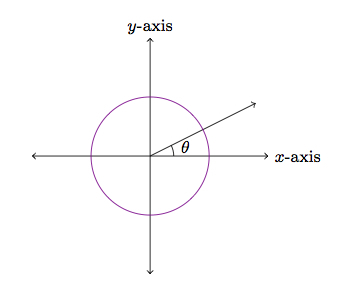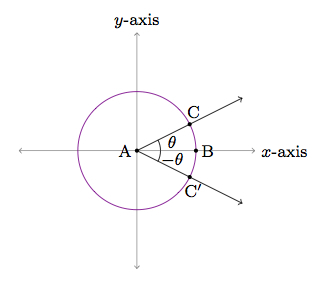# Properties of Trigonometric Functions

Alignments to Content Standards: F-TF.A.2 F-TF.A.4

Below is a picture of an angle $\theta$ in the $x$-$y$ plane with the unit circle sketched in purple:1. Explain why $\sin{(-\theta)} = -\sin{\theta}$ and $\cos{(-\theta)} = \cos{\theta}$. Do these equations hold for any angle $\theta$? Explain.

2. Explain why $\sin{(2\pi + \theta)} = \sin{\theta}$ and $\cos{(2\pi +\theta)} = \cos{\theta}$. Do these equations hold for any angle $\theta$? Explain.

## IM Commentary

The goal of this task is to use the unit circle and rigid transformations in order to establish some fundamental trigonometric function identities. One way to approach part (a) is to use reflection about the $x$-axis to change the sign of the angle $\theta$. Alternatively, students can observe, working with the unit circle, that moving clockwise through $\theta$ radians from $(1,0)$ will give a point with the same $x$-coordinate and the negative of the $y$-coordinate versus moving counterclockwise. Part (b) requires a working knowledge of radian measures. This task complements http://www.illustrativemathematics.org/tasks/1698 which explores the impact of these trigonometric identities on the graphs of sine and cosine.

For a more concrete version of part (a) of the task, the teacher could give students specific values of $\theta$ (such as 30, 45, 60, and 90 degrees) and have the students use the unit circle to calculate the sine and cosine of these angles as well as -30, -45, -60, and -90 degrees. This would align well with F-TF.2.

## Solution

1. Below is a picture of the angle of measure $\theta$ with some points labeled. Also shown is the reflection of this angle about the $x$-axis.As drawn $m(\angle BAC) = \theta$ and $m(\angle BAC^\prime) = -\theta$. We have $C = (\cos{\theta}, \sin{\theta})$. Since $C^\prime$ is the reflection of $C$ about the $x$-axis it has the same $x$-coordinate as $C$ while the $y$-coordinate of $C^\prime$ is the negative of the $y$-coordinate of $C$: $$C^\prime = (\cos{\theta},-\sin{\theta}).$$ On the other hand, by the definition of sine and cosine of signed angles we have $$C^\prime = (\cos{(-\theta)},\sin{(-\theta)}).$$ From these two ways of viewing the coordinates of $C^\prime$ we deduce that

\begin{align} \cos{\theta} &= \cos{(-\theta)} \\ -\sin{\theta} &= \sin{(-\theta)}. \end{align}

The same is true for any angle $\theta$. Reflection about the $x$-axis interchanges the notions of clockwise and counterclockwise. This means that rotating through $-\theta$ radians (for any real number $\theta$) is the same as rotating through $\theta$ radians and then reflecting about the $x$-axis. Rotating through $-\theta$ radians, from $(1,0)$ gives us the point $$(\cos{(-\theta)},\sin{(-\theta)}).$$ Rotating through $\theta$ radians and reflecting about the $x$-axis gives us the point $$(\cos{\theta},-\sin{\theta})$$ and from here we deduce that for any angle $\theta$,

\begin{align} \cos{(-\theta)} &= \cos{(\theta)} \\ \sin{(-\theta)} &= -\sin{\theta}. \end{align}

2. There are $2\pi$ radians in a full rotation of the circle so this means an angle of measure $\theta + 2\pi$ meets the unit circle at the same point as an angle of measure $\theta$. Hence for any angle $\theta$

\begin{align} \cos{(2\pi + \theta)} &= \cos{\theta} \\ \sin{(2\pi + \theta)} &= \sin{\theta}. \end{align}